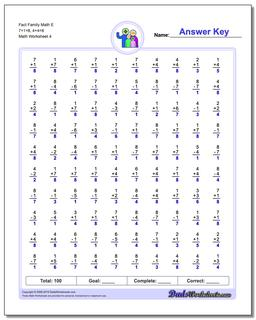One Dad. Four daughters. 9,211 worksheets... and counting!Fact Family Math E 7+1=8, 4+4=8 (Fourth Worksheet)

PropertyValue
DescriptionFact Family Math E 7+1=8, 4+4=8: These math worksheets have 100 addition and subtraction fact family problems and make for a challenging two minute test. (Fourth Worksheet)
Resource TypeWorksheet